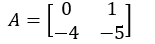Engineering Jobs   »   Quiz: Electrical Engineering 12 Dec 2020

# Quiz: Electrical Engineering 12 Dec 2020

Quiz: Electrical Engineering
Exam: UPPSC-AE
Topic: Miscellaneous

Each question carries 1 mark.
Negative marking: 1/3 mark
Time: 10 Minute

Q1. The starting current and torque of a 3-phase induction motor on direct line starting is 30 A and 300 Nm respectively. What are the corresponding values with star-delta starter?
(a) 30 A and 300 Nm
(b) 30 A and 173.3 Nm
(c) 10 A and 100 Nm
(d) 10 A and 173.3 Nm

Q2. What is the ratio of starting torque and maximum torque of a 3-phase, 50 Hz, 4 pole induction motor for a maximum torque at 1200 rpm?
(a) 0.5
(b) 0.6
(c) 0.421
(d) 0.384

Q3. The system matrix of a linear time invariant continuous time system is given byWhat are the roots of the characteristic’s equation?
(a) -1, -4
(b) -1, -5
(c) -4, -5
(d) 0, -1

Q4. Consider the following types of modulation:

1. Pulse modulation
2. Phase modulation
3. Frequency modulation
4. Amplitude modulation
Which of the above modulation are used for telecasting TV programs?
(a) 1 and 2
(b) 3 and 4
(c) 2 and 4
(d) 1 and 3

Q5. When one transformer is removed from △-△ bank of a 30 KVA transformer, the capacity of the resulting 3-phase transformer corresponding input line voltage?
(a) 17.3 KVA
(b) 20 KVA
(c) 11.5 KVA
(d) 25.9 KVA

Q6. In an induction motor, what is the ratio of rotor copper loss and rotor input?
(a) s
(b) 1/s
(c) (1-s)
(d) s/(1-s)

Q7. The AC bridge used for measurement of dielectric loss of capacitor is
(a) Anderson bridge
(b) Schering bridge
(c) Wien bridge
(d) Hay’s bridge

Q8. In a transformer, Low voltage windings are placed nearer to the core in the case of concentric windings because
(a) it reduces hysteresis loss
(b) it reduces eddy current loss
(c) it reduces insulation requirement
(d) it reduces leakage fluxes

Q9. Reactance relay is used for protection in:
(a) Long transmission lines
(b) Short transmission lines
(c) Medium transmission lines
(d) Both long transmission lines and short transmission lines

Q10. The rms value of wave in figure is